##### Actions

An interference added to the signal during its transmission over a communication channel. More precisely, one says that a given communication channel is a channel with additive noise if the transition function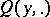of the channel is given by a density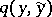,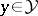,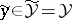(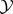and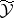are the spaces of the values of the signals at the input and output of the channel, respectively) depending only on the difference, i.e.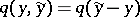. In this case the signal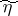at the output of the channel can be represented as the sum of the input signaland a random variableindependent of it, called additive noise, so that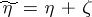.
If one considers channels with discrete or continuous time over finite or infinite intervals, the notion of a channel with additive noise is introduced by the relation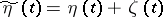, whereis in the given interval,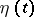,and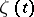are random processes representing the signals at the input and the output of the channel with additive noise, respectively; moreover, the processis independent of. In particular, ifis a Gaussian random process, then the considered channel is called a Gaussian channel.